Journal of Electrical and Electronic Engineering
Volume 4, Issue 3, June 2016, Pages: 44-50

Performance of Superconducting Synchronous Generator for Three-Phase Short-Circuit at Its Terminals

H. Lale Zeynelgil

Department of Electrcal Eng., Electric & Electronics Faculty, Istanbul Technical University, Maslak Istanbul, Turkey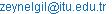H. Lale Zeynelgil. Performance of Superconducting Synchronous Generator for Three-Phase Short-Circuit at Its Terminals. Journal of Electrical and Electronic Engineering. Vol. 4, No. 3, 2016, pp. 44-50. doi: 10.11648/j.jeee.20160403.11

Received: March 24, 2016; Accepted: April 8, 2016; Published: May 7, 2016

Abstract: In this paper, three-phase short-circuit at the terminals of a superconducting synchronous generator is simulated by using MATLAB. In this simulation, a single screened superconducting generator directly connected to power system is considered and two operating cases are investigated: the first case is that the fault is permanent and the second case is that the fault is cleaned by a protection system. To show the effects of this short-circuit and the performance of the superconducting synchronous generator for both cases, the deviations of armature momentary currents, angular speed and field current are plotted. In order to compare the obtained results, the present simulations for the both cases mentioned above are repeated for a conventional synchronous generator having the same rating as considered superconducting counterpart, which is directly connected to power system, and the deviations of the same variables as considered for the superconducting synchronous generator are also given in the paper.

Keywords: Superconducting Synchronous Generator, Screen, Conventional Synchronous Generator, Three-Phase Short-Circuit

Contents

1. Introduction

Electrical energy has very important role for human life and it is essential to supply qualified electrical energy to the consumers. The transient performances of the synchronous generators in the power system are very important for this aspect. Since the requirement of electrical energy increases more and more, the ratings of generating units must be increased and the distribution networks must become relatively complex. However, the ratings of generators are limited due to technological constraints such as electrical, mechanical and transportation problems. Superconducting synchronous generators have various advantages over conventional ones to overcome the maximum rating problem beside improving the transient performance [1-3].

One of the most familiar methods to investigate the transient performance of synchronous machines is the three-phase short-circuit test since the transient current mostly occurs for this fault. When the three-phase short-circuit occurs in a power network, the fault is cleaned by protection system during the generator is on. Following this, very high currents pass through the armature windings. Especially, when there is a three-phase sudden short-circuit at the terminals of a synchronous generator the transient current is much higher than its nominal current [2, 4, 5].

A superconducting synchronous machine consists of a rotating cryogenic superconducting field winding and stationary armature winding at ambient temperature. Superconducting materials are not preferred for armature windings in the stator because it is difficult to use these as alternative current windings . Also, an exceed flux is produce at superconducting field winding, so the iron core in rotor reach to the saturation point and overheat and noisy operation are occurred. Therefore, iron is used only to stator yoke as shield .

There are one or two screens between rotor and stator in superconducting synchronous machines. The screens have two fundamental tasks. One of them is to protect the superconducting field winding form magnetic field deviations and the other is to damp rotor angle oscillations especially for short circuits. Therefore construction and material selection are restraint factors for the screens .

In this paper, three-phase short-circuit at the terminals of a superconducting synchronous generator directly connected to power system is simulated by using MATLAB. Both the cases of the fault is permanent and cleaned are investigated. To show the behaviour of superconducting synchronous generator when there is a three-phase short circuit at its terminals the deviations of armature momentary current, rotor angle, angular speed and field current are plotted for the cases which are considered. Finally, this investigation is repeated for a conventional synchronous generator, which is directly connected to power system in order to compare the behaviours of the superconducting and the conventional synchronous generators for the considered fault.

2. Mathematical Model Used in the Simulation

2.1. Modelling of Single Screened Superconducting Generator

A superconducting synchronous generator having superconducting field windings and single rotor screen is considered, and a three-phase short-circuit at the terminals of the generator is investigated in the simulation. It is assumed that the generator is directly connected to power system. To obtain the mathematical model of the generator, armature windings are assumed to be static and they are transformed to the rotating and orthogonal windings with the same speed as the rotor windings by using Park Transformation [3, 9, 10]. The transformation matrix equation is given as,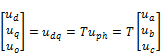(1)

where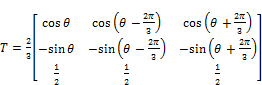(2)

From above expression, the mutual inductances between armature and rotor windings are constant, and thus the mathematical model having constant coefficients is obtained for real superconducting generator, which is a system varying in time.

When the Park transformation mentioned above is applied to flux-current relations for armature windings, the following equations for armature and rotor windings of the machine are given as [9, 10],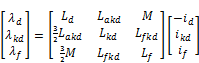(4)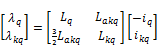(5)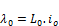(6)

The relations for transformed stator voltages in d and q axes are obtained as,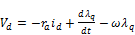(7)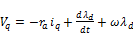(8)

where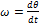.

and transformed relation of the power is obtained as,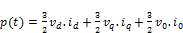(9)

By substituting the relations for stator voltages in d and q axes in Eq. (9), it is obtained instantaneous power as,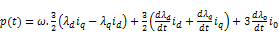(10)

According to above given equation, the instantaneous power consists of shaft power and the variation in stored energy. Thus, the shaft torque is defined as,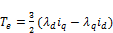(11)

2.2. The Model in Per-unit Values

The per-unit values are used to investigate the performance of power systems. A base value XB is determined to represent any variable X in per-unit values and per-unit value for this variable is defined as,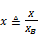(12)

Generally, nominal values of the considered system are used as base quantities. In this respect, the base values for power, impedance, flux and torque are determined, respectively, as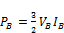(13)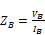(14)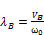(15)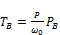(16)

Since the frequency is different for any winding, the base quantities for voltage and current can have different values Therefore, the flux-current relations for d axis in per-unit values are obtained as,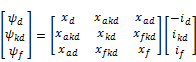(17)

where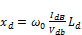(18)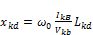(19)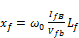(20)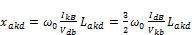(21)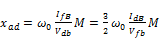(22)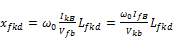(23)

and(24)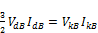(25)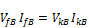(26)

Flux-current relations for q axis are written as similarly to those obtained for d axis as follows: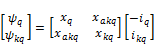(27)

The same base power value is assumed for all of windings since the base values for voltages and currents of armature windings are taken as effective values while those of rotor windings are taken as DC values in the particular relations. If it is assumed that the base values for voltages and currents of all windings are same the mutual inductances between armature windings and, field windings and screen in each axis are equal as,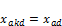(28)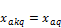Also, the fact that the rotor screen is a thin, continuous shell leads to the shielding constraint: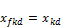(29)

Thus, flux-current relations given by Eqs. (17) and (27) are obtained as [2,9,10],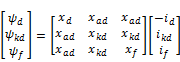(30)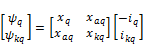(31)

The relations for stator voltages in per-unit values are given as,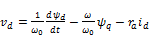(32)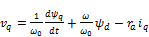(33)

where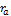is armature windings resistance in per-unit values. The relations for rotor windings in per-unit values are also given as,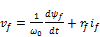(34)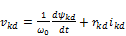(35)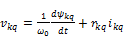(36)

Finally, the relations for rotor dynamics in per-unit values are given as,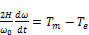(37)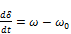(38)

where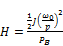(39)

2.3. Simulation Model

In order to investigate the dynamic performance of a superconducting synchronous generator in the case of a three-phase short-circuit at the terminals of the generator, a model containing the differential equations for voltages behind sub-transient reactance are used in this paper [9,10].

The mathematical model used for the simulation model consists of number of ten first order differential equations given as: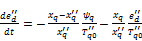(40)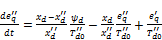(41)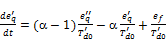(42)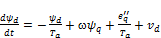(43)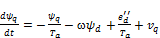(44)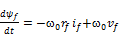(45)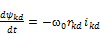(46)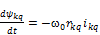(47)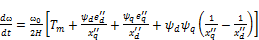(48)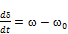(49)

where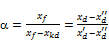(50)

3. Simulation Studies

Three-phase short-circuit is assumed as one of the biggest fault that a generator must withstand. Especially, when short-circuit occurs at the terminals of the generator very high currents pass through armature windings of the generator.

In this paper, three-phase short-circuit at the terminals of a superconducting synchronous generator directly connected to power system is simulated. The parameters for the synchronous generator are given in Table A1 at Appendix . It is assumed that the considered generator has superconducting field windings on rotor and single rotor screen. Then, this investigation is repeated for a conventional synchronous generator directly connected to power system for comparison to behaviours of these generators. The parameters for the conventional synchronous generator are also given in Table A2 at Appendix . For the simulation, a programme is written by using MATLAB and the mathematical models reflecting real behaviour of the system are used to represent both of the generators.

In these simulations, firstly, it is assumed that each machine considered operates in steady state conditions and then a three-phase short-circuit occurs at the terminals of the considered machine at arbitrary time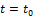. For comparison, steady state operation conditions for the superconducting and the conventional synchronous generators are given in Table 1 and 2, respectively. The strategy of simulation study is same for each case, except the differences in mathematical models of the generators resulting from physical differences between these generators.

Table 1. Steady state operation conditions for superconducting synchronous generator.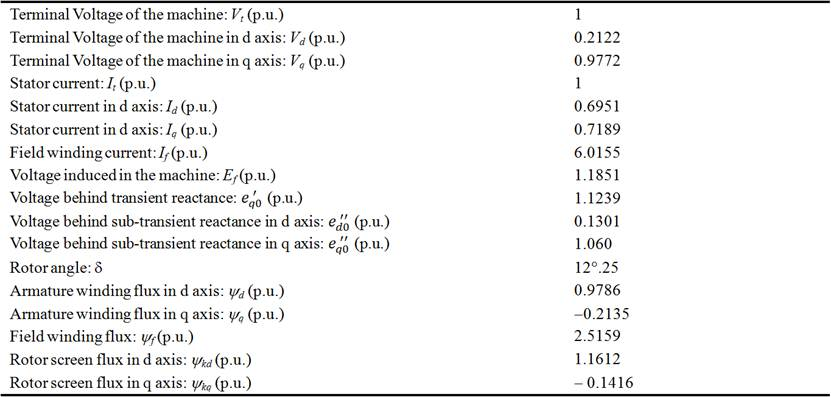Two operating cases for both superconducting and conventional synchronous machines are investigated in this study; the considered short-circuit is permanent and cleaned by protection system at 0.2 seconds. For both cases, to show behaviour of superconducting synchronous generator when a three-phase short circuit occurs at its terminals, the deviations of armature momentary current, angular speed and field current are plotted in the simulation. The graphics for the first case are given in Figs. 1 to 3 and for the second case are given in Figs. 4 to 6.

Table 2. Steady state operation conditions for conventional synchronous generator.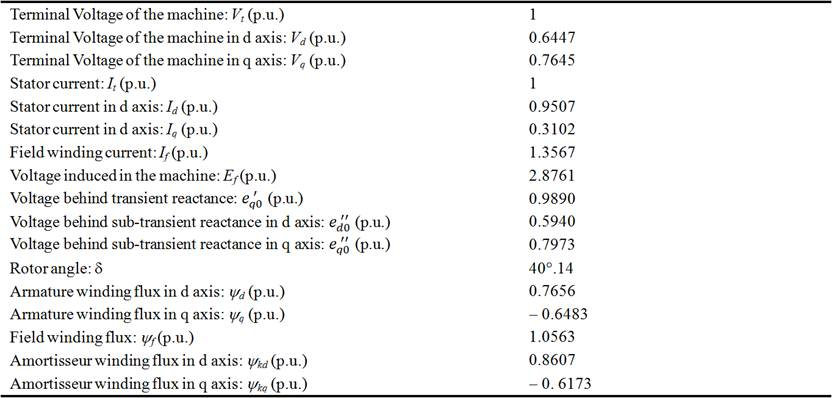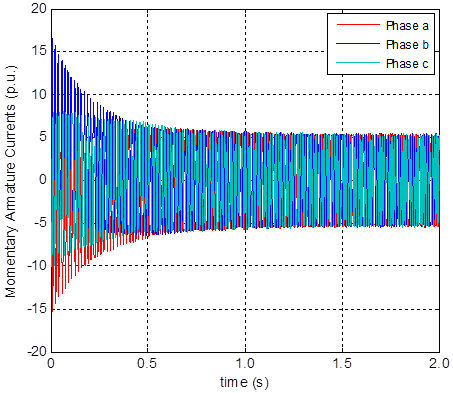Figure 1. Deviations of momentary armature currents for superconducting synchronous generator for the case of permanent fault.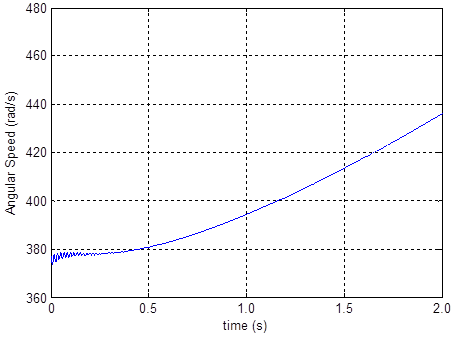Figure 2. Deviation of angular speed for superconducting synchronous generator for the case of permanent fault.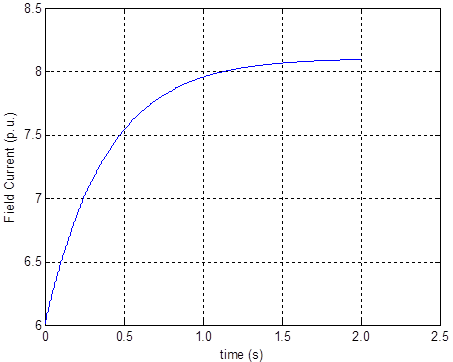Figure 3. Deviation of filed current for superconducting synchronous generator for the case of permanent fault.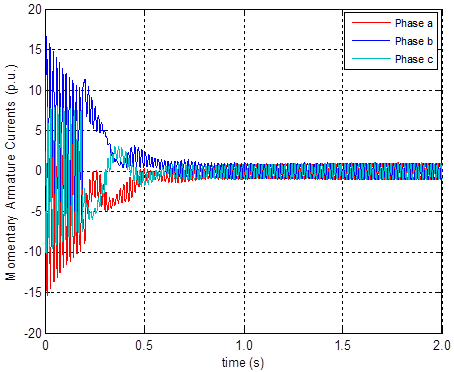Figure 4. Deviations of momentary armature currents for superconducting synchronous generator for the case of removing the fault.Figure 5. Deviation of angular speed for superconducting synchronous generator for the case of removing the fault.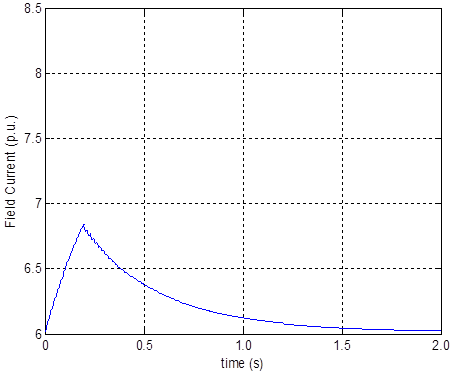Figure 6. Deviations of momentary armature currents for superconducting synchronous generator for the case of removing the fault.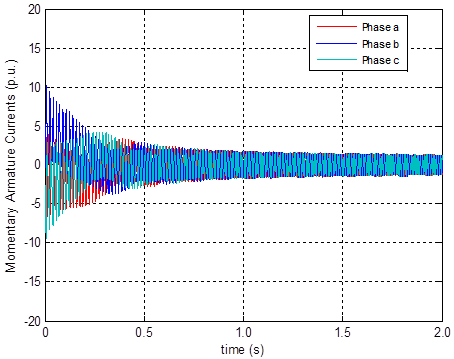Figure 7. Deviations of momentary armature currents for conventional synchronous generator for the case of permanent faultFigure 8. Deviation of angular speed for conventional synchronous generator for the case of permanent fault.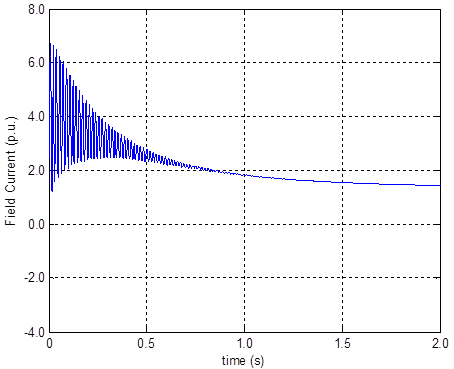Figure 9. Deviation of filed current for conventional synchronous generator for the case of permanent fault.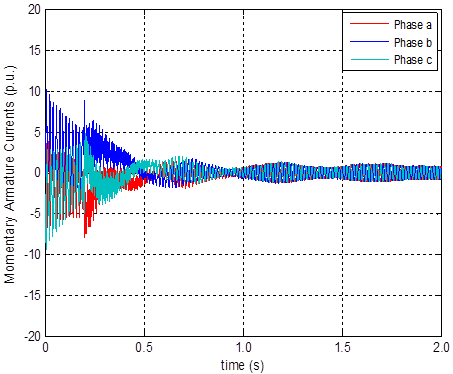Figure 10. Deviations of momentary armature currents for conventional synchronous generator for the case of removing the fault.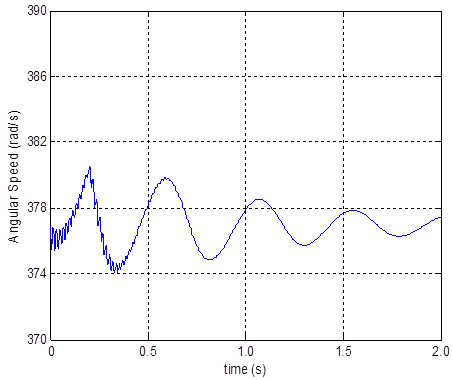Figure 11. Deviation of angular speed for conventional synchronous generator for the case of removing the fault.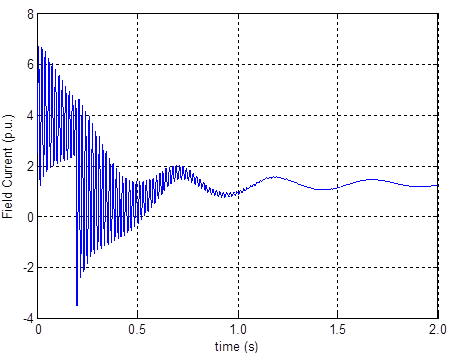Figure 12. Deviation of filed current for conventional synchronous generator for the case of removing the fault.

4. Conclusions

One of the most familiar methods to investigate the transient performance of synchronous generators is three-phase short-circuit test because the most transient current occurs for this fault. In this paper, three-phase short-circuit at the terminals of a superconducting synchronous generator is simulated by means of a simulation programme using MATLAB. In this simulation, a single screened superconducting generator directly connected to power system is considered. To show the effects of the short-circuit the deviations of armature momentary current, angular speed and field current are also plotted in the simulation. Finally, this simulation is repeated for a conventional synchronous generator directly connected to power system for comparison.

Form the results, for the permanent fault case it seen that settling time of the momentary armature currents for superconducting synchronous machine is shorter than those of conventional synchronous machine but steady state currents for superconducting synchronous machine is bigger than those of conventional synchronous machine because synchronous reactance of superconducting synchronous machine is smaller than that of conventional one. On the other hand, momentary armature current rises to 17 p.u. and then oscillates at the interval of (-5;5) p.u. for superconducting synchronous machine. For conventional synchronous machine, these values are obtained as 10 p.u. and (-1.5;1.5) p.u., respectively. Angular speed arises continuously for both machines because the considered fault doesn’t removed. Deviations of field currents for both machines are as expected for the permanent fault case.

For the case that the fault is removed it is seen that the momentary armature currents for conventional synchronous machine cannot settled to steady state values in the simulation period while for superconducting synchronous machine are settled at about 1.0 seconds. For deviations of angular speeds and field currents for both machines are obtained similar results to deviations of momentary armature currents. So, angular speed for conventional synchronous machine cannot settled to steady state values in the simulation period while for superconducting synchronous machine are settled at about 1.35 seconds, and deviations of field currents for conventional synchronous machine cannot settled to steady state values in the simulation period while for superconducting synchronous machine are settled at the end of the simulation period.

Finally, from the simulation results it is seen that the superconducting synchronous machine has more stability than the conventional synchronous machine for a three-phase short circuit at its terminal at both cases of the fault is permanent and cleaned by protection system. Especially, it is clearn that the superconducting synchronous generator is superior to the conventional one in the case of removing the fault.

On this subject, it can be investigated a stability analysis can be made by using equal area criterion. Also, a similar investigation can be carried out for a double-screened superconducting synchronous generator.

Appendix

Table A1. Parameters for superconducting synchronous generator used in the simulation.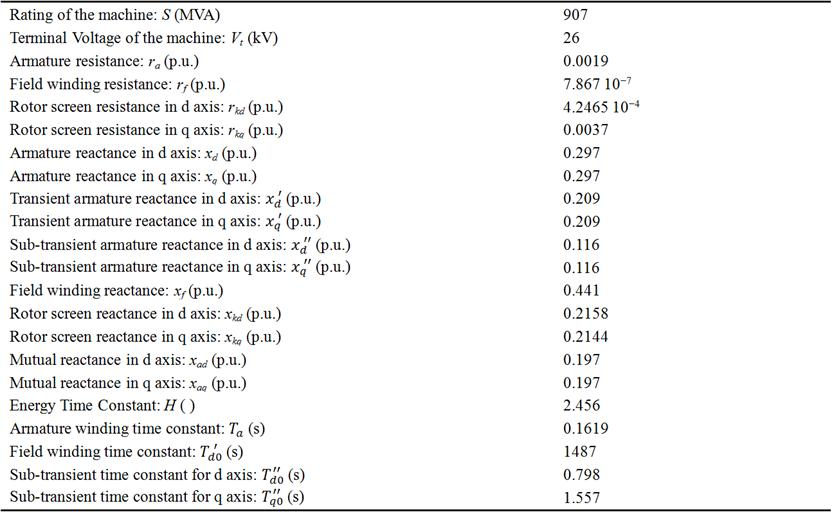Table A2. Parameters for conventional synchronous generator used in the simulation.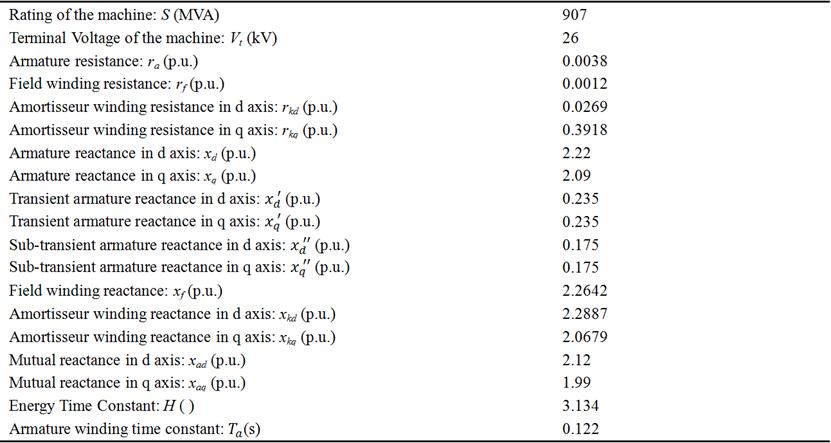References

1. Grant PM (1997) Superconductivity and Electric Power: Promises, Promises ... Past, Present and Future, IEEE Trans. on Applied Superconductivity, 7(2): 112-113
2. Umans SD (2009) Transient performance of a High-Temperature-Superconducting Generator, IEEE Electric Machines and Drives Conference, 451-457
3. Bozdag HO (2011) Simulation of Three-phase Short-circuit at the Terminals of Superconducting Synchronous Generator, M.Sc Thesis, Istanbul Technical University, Institute of Science and Technology
4. Liu X, Liu D, Huang Y (2008) Simulation of Three-phase Short-circuit at the Terminals of Synchronous Machine, ICEM, 115-117
5. Shenkman, Arieh L. (2009) Transient analysis of Electric Power Circuits Handbook", Springer
6. Goddard, K. F., Kukasik, B., Sykulski, J. K. (2009) Alternative designs of High-Temperature Superconducting Synchronous Generator, IEEE Applied Superconductivity, .19(6): 3805-3811
7. Kalsi, Swarn S. (2002) Development status of Superconducting rotating machines" IEEE Pes Meeting
8. Furuyama, M., Kirtley, J. L., 1975, "Transient Stability of Superconducting Alternators", IEEE PAS-94, 320-328
9. Kirtley JL (1993) Large System Interaction of Superconducting Generators, IEEE Proc. on Power Systems, 81(3): 449-461
10. Kirtley JL (1981) Armature Motion Damping of High Temperature Superconducting Generators, IEEE Tras.. on PAS, 100(6): 2870-2879

 Contents 1. 2. 2.1. 2.2. 2.3. 3. 4.
Article ToolsAbstractPDF(301K)